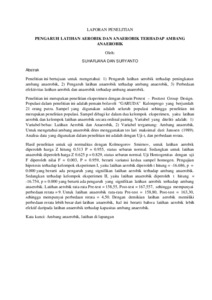# PENGARUH LATIHAN AEROBIK DAN ANAEROBIK TERHADAP AMBANG ANAEROBIK

Suharjana and Suryanto (2004) PENGARUH LATIHAN AEROBIK DAN ANAEROBIK TERHADAP AMBANG ANAEROBIK. [Experiment/Research] (Submitted)Preview
Text
pengaruh_latihan_aerobik_suharjana_2004.pdf

Download (10kB) | Preview

## Abstract

This research is aimed at demonstrating: 1) the effects of aerobic exercise towards the increase of anaerobic threshold, 2) the effects of anaerobic exercise towards the anaerobic threshold, 3) the difference in effectiveness of aerobic and anaerobic exercise towards anaerobic threshold. This is an experimental study using Pretest – Posttest Group Design. The populations involved in this study were 21 volleyball players of GARUDA from Kulonprogo regency, Yogyakarta. The samples were all players of the population so that it is categorized as population research. The samples were divided into two experimental groups which were aerobic and anaerobic groups using ordinal-pairing approach. The variables were: 1) independent variables: Aerobic and Anaerobic Exercises, 2) the dependant variable: anaerobic threshold. To determine anaerobic threshold, the researcher used running tests from Janssen (1989). The data were analyzed using T-test and mean variation. The results of normality tests using Kolmogorov Smirnov for aerobic exercise, Z count 0.513 P = 0.955, the status of spreading was normal while Z count for anaerobic exercise was 0.625 p = 0.829, the status of spreading was normal. Homogeneity tests using F-tests resulted in F = 0.003, P = 0.959, meaning that the variance of the samples was homogenous. The hypothesis test to experimental group I which was the group of aerobic exercise resulted in t count = -16.686, p = 0.000 which implied that there was significant influence of aerobic exercise towards anaerobic threshold and one to experimental group II which was the group of aerobic exercise resulted in t count = -16.754, p = 0.000 which implied that there was significant influence of aerobic exercise towards anaerobic threshold. The mean pre-test was 58.55, Post-test was 167.557, so that the mean difference was 9. For the anaerobic exercise, the mean of Pre-test was 158.80 and Post-test was 163,30, so that the mean difference was 4.50. Thus, the aerobic exercise had bigger mean differences than anaerobic exercise which implies that aerobic exercise was more effective than anaerobic in relation to the capacity of anaerobic threshold. Keywords: Anaerobic threshold, field exercise

Item Type: Experiment/Research OlahragaOlahraga > Pendidikan Jasmani Kesehatan dan Rekreasi FIK Admin 05 Jul 2012 06:50 05 Jul 2012 06:50 http://eprints.uny.ac.id/id/eprint/1717

### Actions (login required)View Item